#### The number of scouts in a school is depicted by the following pictograph: Class Number of Scouts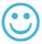= 10 Scouts VI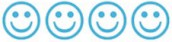VII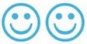VIII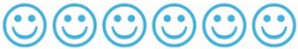IX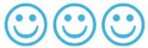XObserve the pictograph and answer the following questions:(a)    Which class has the minimum number of scouts?(b)    Which class has the maximum number of scouts?(c)    How many scouts are there in Class VI?(d)    Which class has exactly four times the scouts as that of ClassX?(e)What is the total number of scouts in the Classes VI to X

(a) Class X has a minimum number of scouts.
(b) Class VIII has the maximum number of scouts.
(c) There are 40 scouts in Class VI.
(d) Class VI has exactly four times the scouts as that of Class X.
(e) Total number of scouts in Classes VI to X is 160.